# A Scheme for Measuring Anisotropy of One‑Way Light Speed.

by David Bryan Wallace
Cape Coral, Florida, USA
Posted to academia.edu 2015-06-11, retitled and revised 2020-04-05.
Posted to Vixra 2020-04-05

### Introduction

The relativity theory of Albert Einstein receives praise as a great scientific achievement. Although it is said to have been consistently confirmed, not all aspects of the theory have been confirmed. The isotropy of one‑way light speed has not been confirmed.

Although special relativity is associated by many with the Michelson-Morley experiment, it was not an explanation of any empirical result. Einstein claimed that when he wrote his special relativity paper, he was unfamiliar with the Michelson Morley experiment except the conclusion that Earth appeared to have zero velocity relative to the luminiferous ether.

The experiment of Michelson and Morley involved no clock and no relative velocity between observer and observed phenomena. It is well explained by George Francis FitzGerald's conjecture that length contraction offsets Abraham Michelson's anticipated lengthening of light's round trip time. Einstein's thinking involved a gedanken experiment about timing a light signal between two clocks and in that is unlike the Michelson-Morley experiment.

Einstein presented special relativity as a deduction based on two postulates, and he said his motivation was a firm belief that there was no reality corresponding to the notion of absolute rest. This belief is open to challenge.

The Postulates of Special Relativity

I. The same laws of electrodynamics and optics will be valid for all frames of reference for which the equations of mechanics hold good.
II. Light is always propagated in empty space with a definite velocity c which is independent of the state of motion of the emitting body.

Together these two postulates have been taken to imply that the speed of light is isotropic in every inertial frame of reference, thus denying a basis in reality for the notion of absolute rest. Yet, the empirical confirmation of the isotropy of light speed has been limited to round‑trip average speed of light, not one‑way speed. International standards now establish by definition that the round‑trip average speed of light is the constant c with respect to any inertial frame of reference, standarizing length measure in any inertial frame interferometrically by the time of a round‑trip of light, length = ½ c × time‑round‑trip. When one assumes time‑forward = time‑back, as special relativity theory requires for its synchronization of clocks using light signals, it vitiates timing the one‑way speed of light between the synchronized clocks. One‑way speed of light, has never been measured. However, with a bit of finesse, as will be shown, today's atomic clocks make one‑way light speed measurable without the clocks being synchronized.

There is reason believe that when relativity's claim of one‑way light speed isotropy is finally tested, it will fail. The Sagnac effect suggests there is anisotropy of one‑way light speed. Astronomical observations also support the expectation that the frame of reference in which one‑way speed of light is isotropic is unique. At rest in such a unique frame of reference is worthy of being deemed absolutely at rest; that unique frame may be called the aether frame.

If absolute rest has no basis in reality the one‑way speed of light will be the same for any evacuated light path independent of orientation for every frame of reference. On the other hand, dependence on orientation would demonstrate a real basis for the notion of absolute rest. This paper presents not only a way to detect which hypothesis pertaining to isotropy of one‑way light speed is tenable, but also a way to measure our absolute velocity relative to the aether. Like the Robertson and Mansouri-Sexl systems, it features a unique inertial rest frame in which one‑way light speed is isotropic. Unlike them it honors the ISO definition of length for establishing local spatial coordinates, thereby keeping round-trip average light speed equal to the defined constant c for every local frame. As in FitzGerald's conjecture, lengths and proper spatial coordinates in moving inertial frames are contracted relative to the rest frame.

### Part 1: A Realizable Test of the Isotropy of Light Speed

Let us devise a test of whether absolute rest has a basis in reality or not.

### Assumptions.

Taking care throughout that we do not assume our conclusion, we shall, for the sake of argument, adopt the conjecture of FitzGerald in this discussion. The fraction of light speed of an inertial frame's velocity relative to the conjectured aether frame is designated β. One time scale shall serve all frames; differences of clock rate are ascribed to clock peculiarities, not to time itself. Length measure is based on the interval of time required for light to complete a round‑trip. The proper length of molecular bonds is fixed; so light's round‑trip between bond ends is of constant duration. To maintain this constant time interval, molecular bonds in motion span a lesser distance than the same bonds at rest, (distance measured in the conjectured aether frame.) The contraction is by a factor (1−β2) parallel to the motion and by (1−β2)½ perpendicular to the motion. These ratios are derived below, and were also derived by Michelson and Morley, and by Einstein in his special relativity paper before a hocus-pocus in the name of “substituting for x′ its value,” after which he uses Lorentz transformations. The same contraction ratios are expected for solid bodies, so spaces between unconnected solid bodies will appear greater relative to the moving local frame than they do to the rest frame.

### Experimental Setup.

Consider an experimental inertial frame with a non‑zero absolute velocity β⋅c with respect to the conjectured aether frame. [note: Boldface is used for the vector value, if not boldfaced, the scalar magnitude is meant.] The experimental frame's absolute velocity is to be determined. Suppose we fix two stable accurate atomic clocks sufficiently far apart and eliminate refraction by evacuating the direct light path between the clocks. Let a light signal be sent forward from the first clock to the second clock where it is reflected back to the first, with times of these events noted according to the respective clocks. Several trials are executed with differing orientation of the line between the clocks. In the example below the change of path orientation is effected by Earth rotation. The clocks need not be synchronized, but must measure time at the same rate throughout all trials.

We shall be interested in:

1. beta, β = v/c, velocity of the experimental frame ÷ speed of light,
alternatively expressed as its vector coordinates, (β, α, δ), where alpha and delta are, respectively, right ascension and declination in the celestial coordinate system.
2. For convenience, we define γ = 1/(1−β2)½.
3. time‑forward, the time taken by light to travel from the first clock to the second clock, possibly including a synchronization error.
4. time‑back,the time taken by light to travel from being reflected at the second clock back to the first clock, possibly including a synchronization error.
5. time‑difference = time‑forwardtime‑back, possibly including a double synchronization error.
6. sigma, σ = time‑sum = time‑forward + time‑back, (time‑round‑trip, no synchronization error.)
7. rho, ρ = time‑difference ÷ time‑sum, (a ratio strictly between one and minus one if, relative to any frame of reference, clocks are synchronized.)
8. phi, φ, the angle between the velocity, β, of the experimental frame and the line determined by the two clocks.

The measure of the angle φ is frame of reference dependant. Accordingly, subscript zero φ0 will denote aether frame value, and subscript beta φβ will denote experimental frame value.The aether frame vector difference c − β⋅c of the velocity of the light signal and the velocity of the experimental frame will have the magnitude c⋅(1−β⋅cosφ0), the same for any evacuated light path the same angle φ0 from β. If the clocks be absolutely synchronized, i.e. synchronized with respect to the aether frame, then perpendicular to β the ratio ρ = time‑difference ÷ time‑sum must be zero, because time‑difference must be zero. However, for light path orientations at other angles φ from β non-zero ratios ρ are expected, and parallel to β the maximum ρ is expected. In contrast, if the special relativity postulates are compatible and absolute rest is meaningless, ρ = 0 always.

Figure 1 idealizes a trial of the experiment in aether frame scale. The two clocks, synchronized in the aether frame, move to the right at speed βc, the points A, B, and C are the fixed aether frame locations where three events occur: at A, the first clock emits a light signal at time_1 as it passes through point A; at B, the second clock reflects the light signal at time_2; at C, the light signal and the first clock arrive together at time_3. The point D is the location of the first clock at time_2. So we have time intervals t1 = time_2 − time_1 and t2 = time_3 − time_2. The angle between the line determined by the two clocks and the velocity v = β⋅c of the experimental frame is labeled φ.

### Derivations.

Let us now derive a equations for our variables of interest and the generalized transformations for any value of φ. Then, we will see the very ratios derived by Michelson and Morley for φ = 0 and φ = ½π.

The cosine law and quadratic formula yield solutions for t1 and t2 in the triangles ADB and CDB respectively. First, t1 from triangle ADB in equations (1.1) through (1.3):(1.1) cosine law for ΔADB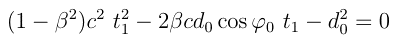(1.2) eq 1.1 in standard quadratic form(1.3) quadratic formula solution

Then, t2 from triangle CDB in equations (1.4) through (1.6):(1.4) cosine law for ΔCDB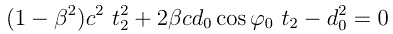(1.5) eq4 in standard quadratic form(1.6) quadratic formula solution

Now we see the time-difference, the time-sum, and the ratio ρ.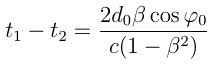(1.7) time-difference(1.8) time-sum(1.9) ratio

The experimental frame observer judges length by round-trip time of light, so dβ = ½c(t1 + t2). The equations for tranformation of lengths between moving and aether frames are:(1.10) length transformation,      [recall: γ = 1/(1−β2)½.]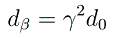(1.11) parallel, sin 0 = 0(1.12) perpendicular,        sin 90° = 1
The spatial distance appears greater in the moving frame because the local measuring sticks are contracted.

When the length transformations are applied to trigonometric functions, it turns out that ρ, serendipitously, is a quite simple function of φβ:(1.13) ρ(φβ)

### Expectations and Analysis.Polar coordinate plots of ρ as a function of φβ for constant β appear in figure 2.

For clocks absolutely synchronized, the two dimensional plot is a circle that intersects the origin at φ = ½⋅π, and the diameter from the origin will equal β. The plot for φ ∈ [0, ½⋅π] coincides with the plot for φ ∈ [½⋅ππ].

A synchronization error would produce a ρerror less than β if for some φ the clocks had been locally synchronized rather than being absolutely synchronized. In that case, the plot will separate into two loops; the 2D plot is a limaçon. The magnitude of the error ρerror is half the distance between the two absolutely maximal intersections with the symmetry axis, and β will be the midpoint between the same two points. Rotation about the symmetry axis yields a three-dimensional plot.

Shown below in figures 3 and 4 are three-dimensional celestial coordinate plots of ρ = β⋅cos(φβ) for every possible direction. The surfaces have symmetry axes through the origin parallel to β. In the absence of synchronization error, β is the point (ρ, α, δ) of the sphere farthest from the origin, possibly deduced from a minimum of four independent data points. A constant synchronization error would add a fixed ρerror to the magnitude of each data point, presenting an expanded spheroidal surface for ρraw = ρtrue + ρerror of one sign and a contracted surface for ρraw of the other sign. There would remain an intersection at the origin ρraw = 0. The two unknowns, β and synchronization error, ρerror, can both be determined from the full surface plot. The point midway between the surface's two absolutely maximal points is β. The value of ρraw for φ = ½⋅π will be ρerror, which is also half the distance between the maximal points. The full plot can be reproduced from as few as five data points.

As Earth turns, an earth-bound horizontal light path of fixed length not parallel to the earth's rotational axis will present a cone of orientations of like declination δ that includes (1) a path of maximal ρraw at φmin, (2) a path of minimal ρraw at φmax, and (3) equal φ pairs with equal ρraw. This is a pattern of bilateral symmetry about a plane parallel to both β and the earth axis. A lack of synchronization does not alter the orientation of the symmetry plane provided the synchronization error is constant. Figures 3 and 4 show a dark trace for such a conical sample with declination δ = 45° and ρerror = 0.0003 on a surface representing all possible orientations; in figure 3 β = (0.001, α, 32.7°), and in figure 4 with β = (0.001, α, −24.6°).

How accurate must clocks be to make a decisive test? Let us suppose a test site situated at the Bonneville Salt Flats (Lat. 40.736556, Long. -113.411537) with path ninety kilometer long and forward direction about 19° east of true north to give a constant 45° declination. The relative velocity of the endpoints of the path would be less than 5 meter per second. The value of σ is 0.6 millisecond. Further, suppose β = (β, α, δ) = (0.001, 10°, −34°). The expected 0.00117 range of ρ, from 0.001⋅cos(169°) = -0.00098 to 0.001⋅cos(79°) = 0.00019, implies a one-way timing range of 0.35μs. Nanosecond timing accuracy would resolve this range into 350 parts, enough for a decisive test.

 ρraw = ρtrue + ρerror (1.13) error of second clock time relative to first clock = ½⋅σ⋅ρerror (1.14)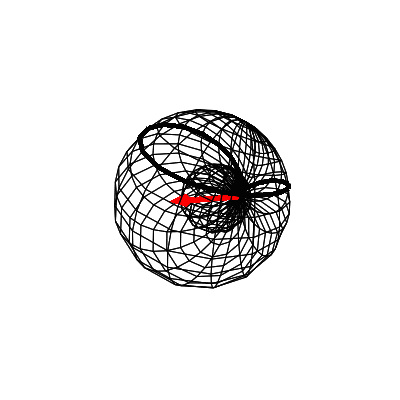(figure 3)β = (0.001, α, 32.7°)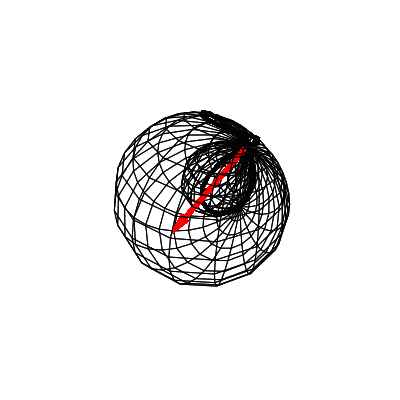(figure 4)β = (0.001, α, −24.6°)

Due to Earth's motions an earth-bound experiment site has inconstant β, so the determination of β must be referred to a specific time and place. In the final analysis, data must be adjusted for deviations from this β. For clarity, the preliminary exposition above neglected the variations of velocity due to Earth motion.

The ranges of significant known oscillatory motions that are superimposed on the unknown β include up to 60,000 meter per second (0.0002 light speed) due to the earth-solar orbit, up to 920⋅cos(latitude) meter per second due to earth rotation, and up to 25 meter per second due to the earth-lunar orbit. The effect of these motions can be removed from the raw data by subtracting the parallel component of vdeviation/c. For example, if the raw datum (ρ=0.000010, α=75°, δ=45°) is taken when the motion differs from the sought unknown β by vdeviation/c = (0.000003, 15°, 50°) = (light speed fraction, α, δ), one finds adjusted datum magnitude (direction unchanged) ρadjusted = 0.000010 − 0.000003⋅cosine(angle difference) = 0.000010 − 0.000003⋅((cos(75°−15°)+cos(90°−45°)⋅cos(90°−50°))/(sin(90°−45°)⋅sin(90°+50°)) = 0.0000031, where the spherical law of cosines for sides has been used to find the cosine of the angle difference.

Ultimately, considering the much longer light paths achievable with a space-based test, an earth bound test may be deemed uninteresting. A space based test would involve variable path lengths, but ρ is independent of path length after all.

### Part 2: Revising Relativity Theory to Accommodate an Absolutely Stationary Frame of Reference

If it is found that isotropic light speed is not an attribute of every inertial frame of reference, then relativity theory needs revision, and a caveat is needed with the principle of invariance: "In any inertial frame of reference the laws of physics are the same for all phenomena except those that depend on isotropy of the one-way speed of electromagnetic propagation."

The Michelson Morley experiment involved no clocks and so reveals no such thing as time dilation. Neither did it involved any relative motion of observer and observed phenomena. Aside from its ad hoc correspondence to the conclusion, Einstein's relativity theory does not account for it.

The apparent null of the Michelson Morley experiment is explained by length contraction of moving solids being precisely such as to produce constancy of locally determined interferometric length, (1−β2) parallel to β and sqrt(1−β2) perpendicular thereto. This should not be a great surprise as it is implicit in the use of interferometric standards for length measure. A curious feature of Einstein's special relativity paper is that these were the contraction ratios he derived before an unexplained switch to the Lorentz transformations. The Lorentz transformations are incorrect because they fail to recognize contraction transverse to motion. Equations 2.1 through 2.4 show correct transformations between rest frame coordinates (x0, y0, z0) and moving frame coordinates (xβ, yβ, zβ), where x-axes are parallel to β, origins coincide at t0 = tβ = 0 and y- and z-axes respectively are parallel. Figure 2.5 expresses these transformations in matrix form.

### Transformation of Coordinates

t0 = tβ (2.1)(2.2a)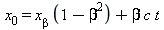(2.2b)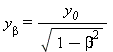(2.3a)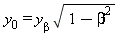(2.3b)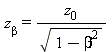(2.4a)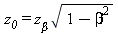(2.4b)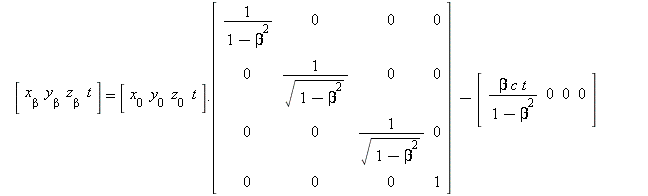(2.5a)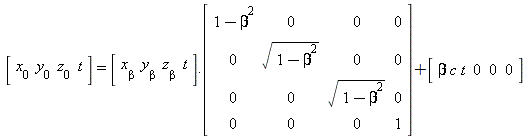(2.5b)

The variant of relativity being offered here is essentially classical or Galilean relativity modified by the inclusion of contraction of solids induced by absolute velocity. Every moving coordinate system is to be set up using the contracted length measure proper to the system, corresponding to the defined interferometric standard applied locally. Time is well defined and shared by all frames of reference. A distinct variant of time called "local time" may be defined for a specific inertial frame of reference. If clock rate is affected by motion or gravitation, that shall be regarded as a peculiarity of the clock, not a peculiarity of time.

A solid rod, which at absolute rest would have length l, contracts when moving. If moving at fraction of light speed β with the angle φ0 (φβ in the moving frame) between β and the axis of the rod, the absolute span s0 of the rod is given by. The proper length of the rod, measured in the inertial frame of the rod itself, remains l.

In the moving frame we find the angle φ transformed,; ifthen.

In the moving system, a point at absolute rest (at rest in the rest frame) will have velocity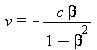. On the other hand, a point at rest in the moving system will in the rest frame have velocity βc. Time in all frames of reference is the same as in the rest frame and might be called universal time or absolute time. However, for some applications it will be preferable to ignore β and synchronize clocks with light signals assumed to take the same time each way; such synchronization is outside the practices of our modified relativity and shall be called "local synchronization" giving rise to "local time." If a moving system has its x-axis parallel to β and local time at the origin is equal to universal time, then local time at other points is related to universal time by tuniversal = tlocal + β x/c. Importantly, this relationship allows synchronization to absolute time by light signals once β is known.
 tuniversal = tlocal + β x/c (2.6)

### Transformation of Measure

tan(φ)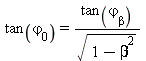(2.7a)

(2.7b)

cos(φ)(2.8a)

(2.8b)

sin(φ)(2.9a)

(2.9b)

absolute span of moving length(2.10a)

(2.10b)

ψ dihedral angle between two angles φ1 and φ2

ψ0 = ψβ

(2.11)

θ angle between the vectors β1 and β2 of two inertial frames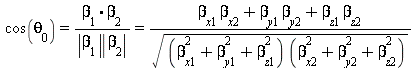(2.12)

### Part 3: Stellar Aberration

An observer's motion can affect the percieved right ascension and declination of a star's position. This has been expressed as an angular difference relative to the direction perceived by an observer motionless relative to the solar barycenter. The purpose here is to find the moving observer's stellar aberration αβ relative to an observer absolutely at rest.

The angular aberration αβ, (not to be confused with right ascension α,) is always from the rest frame position of the star toward the direction of the observer's β. Analysis of this phenomenon for an observer of known β begins by transforming the angle φβ observed for a star to the angle measure of the rest frame φ0 observed, see equation 3.1. The sine of the rest frame measure of the aberration equals β⋅sin(φ0 observed), as shown in figure 5. The goal of finding αβ = φ0 true  −  φβ observed is reached in equations 3.3 through 3.7.(3.1)[applying 2.9a](figure 5)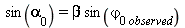(3.3)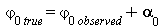(3.4)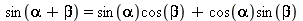(trigonometric identity)(3.5)(3.6)(3.7)

### References

 Wallace, David Bryan, "Improving the Definition of Simultaneous Events" Academia.edu (2013) https://www.academia.edu/7273747/Essay_Improving_the_Definition_of_Simultaneous_Events

 de Sitter, Willem, “Ein astronomischer Beweis für die Konstanz der Lichgeshwindigkeit," Physik. Zeitschr, 14, 429 (1913). “A proof of the constancy of the velocity of light,” Proceedings of the Royal Netherlands Academy of Arts and Sciences 15 (2): 1297-1298, online in English http://www.shadetreephysics.com/desit-1e.htm..

 Relativists overlook that some observations are independent of frame of reference. In the case of de Sitter’s observations, the ratio of the distance between the stars of a double star pair and the distance of that double star pair from Earth is as unchanging in one frame as in another. Likewise, the ratio of the time taken for light emitted by the star when receding to the time taken by light emitted from the star when approaching is also frame of reference independent. The implication is that relative to any frame of reference including the frame of the star, the speed of light emitted is constant relative to a frame of reference other that of the star itself and is not constant relative to the star.

Actually, there is an error in de Sitter’s reasoning, but correcting that error only makes his proof more compelling. See the animated portrayal of ballistic theory as it pertains to de Sitter’s proof. Think of each emitted dot as a wave crest and notice how wavelength becomes dependent on distance from the star, even sometimes vanishing (infinite frequency). De Sitter overlooked this effect taking wavelength as being Doppler shifted only. The overlooked (hypothetical) effect would have been vastly greater than the Doppler effect de Sitter had in mind. No such effect is seen.

De Sitter’s proof is a compelling refutation of SRT. It is symptomatic of relativists’ sloppy thinking that they hail it as a verification of relativity.